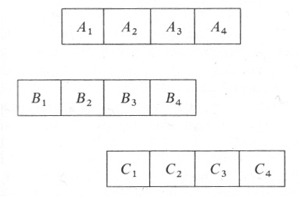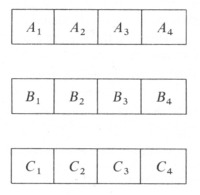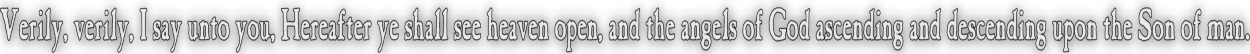None:
Polyps:
Strongs:CreationismGreeks, Time And Commensurability Commensurability is often described in terms of length. Two lengths are commensurable if there is a ratio between the two of whole measure. If one length is two units and the other say 45/37 the length of the first, then there is a correspondence of 74 of the first to 45 of the second that are equal measures of the unit. Simple as it sounds from our understanding of fractions this was a big deal to the Greeks. Why this was so important is that the relationships are independent of the units used. The relationship is the same for units of inches, metres or miles. Nevertheless the thing measured must be the same, or else just numbers. One couldn't compare volume to speed etc. Likewise there are numerous irrational numbers that are not commensurable: Pi, the ratio of a circle's diameter to it's circumference is an example. It was a point of debate that there was no unit of choice that would make such numbers commensurable. Also, there were relationships (such as like the distance covered by a body at a certain speed), that are not entirely independent, but are proportional and when examined with the idea of commensurability many paradoxes arise that show that these measures (speed, distance) if commensurate, would allow the paradox that motion would be impossible. Commensurability of these using the unit of time (as with inches) to relate speed and distance is arguable from the view that the 'present' is the constant, and not the 'length' of time passing. Here follows a few of those paradoxes. (Taken from Carl B. Boyer's book "A History of Mathematics") ..."Aristotle described a Pythagorean point as "unity having position" or as "unity considered in space." It has been suggested that it was against such a view that Zeno propounded his paradoxes, of which those on motion were cited most frequently. As they have come down to us, through Aristotle and others, four of them seemed to have caused the most trouble: (1) the Dichotomy, (2) the Achilles, (3) the Arrow, and (4) the Stade. The first argues that before a moving object can travel a given distance, it must first travel half this distance; but before it can cover this, it must travel the first quarter of the distance; and before this, the first eighth, and so on through an infinite number of subdivisions. The runner wishing to get started, must make an infinite number of contacts in a finite time; but it is impossible to exhaust an infinite collection, hence the beginning of motion is impossible. The second of the paradoxes is similar to the first except that the infinite subdivision is progressive rather than regressive. Here Achilles is racing against a tortoise that has been given a head start, and it is argued that Achilles, no matter how swiftly he may run, can never overtake the tortoise, no matter how slow it may be. By the time that Achilles will have reached the initial position of the tortoise, the latter will have advanced some short distance; and by the time Achilles will have covered this distance, the tortoise will have advanced somewhat further; and so on the process continues indefinitely, with the result that the swift Achilles can never overtake the slow tortoise.    The Dichotomy and the Achilles argue that motion is impossible under the assumption of the infinite sub divisibility of space and time; the Arrow and the Stade on the other hand, argue that motion is equally impossible if one makes the opposite assumption - that the sub divisibility of space and time terminates in indivisibles. In the Arrow, Zeno argues that an object in flight always occupies a space equal to itself; but that which always occupies a space equal to itself is not in motion. Hence the flying arrow is at rest at all times, so that it's motion is an illusion.    Most controversial of the paradoxes on motion, and most awkward to describe, is the Stade (Or Stadium), but the argument can be phrased somewhat as follows. Let A1, A2, A3, A4 be bodies of equal size that are stationary; let B1, B2, B3, B4 be bodies, of the same size as the A's, that are moving to the right so that each B passes an A in an instant - the smallest possible length of time. Let C1, C2, C3, C4 also be of equal size with the A's and B's and let them move uniformly to the left with respect to the A's so that each C passes each A in an instant of time. Let us assume that at a given time the bodies Occupy the following positions:Then after the lapse of a single instant - that is, after an indivisible subdivision of time - the positions will be as follows:It is clear, then that C1 will have passed two of the B's; hence the instant cannot be the minimum time interval, for we can take as a new an smaller unit the time C1 takes to pass one of the B's.    The Arguments of Zeno seem to have had a profound influence on the development of Greek Mathematics, comparable to the discovery of the incommensurable, with which it may have been related. Originally, in Pythagorean circles, magnitudes were represented by pebbles or calculi from which our word calculation comes, but by the time of Euclid there is a complete change in point of view." - (taken from "A History of Mathematics" Chapter V section 11, by Carl B. Boyer, Wiley International Edition) Sometimes however, efforts similar to this early Greek use of time as a unit of commensurability has real world application. Astronomy near grounded to a halt with observed disparity between the magnitude of spectra of starlight and the modelled predictions. It was discovered that a substitution of E=hf, (E energy, f frequency, and h a constant) within the model provided an inter-relation of E and f that accurately predicted the detected spectra when taken into account. So what use is this to understanding dialectic logic? Simply the assumption that everything logical is also commensurable. The inversion of thesis/antithesis to the inconsistency between the two remaining balances of the four (as in synthesis) leads to the method using those two outcomes (incompatible with each other) as commensurable - the thesis/antithesis from which the two negations arise must be "married" as commensurate if the method is to be held correct. The method then takes two opposing factors that may never agree and itself as the conjunction, supplies a "common measure" where the method is itself synthesis, commensurating the opposition of thesis/antithesis. Likewise any two opposites birth two new opposites that birth the starting pair: we have the stance of "as above, so below" that is intrinsically deceptive and a tenet of satanism, direct from the serpent. Being able to quickly adapt the principle and give the appearence of commensurability with the method is the very heart of the work of satan, deception.   Continue To Next Page Return To Section Start Return To Previous Page'